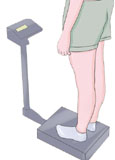# BMR Measurement

### Measurement of BMR

Since BMR measurement is done in terms of heat output, the process of measurement of BMR is often called calorimetry. It is of two types—
i) direct calorimetry
ii) indirect calorimetry.

#### Direct calorimetry of BMR measurment

This is based on direct BMR measurement of heat evolved from the body of the subject. A specially prepared heat-proof chamber known as Atwater-Benedict's respiration calorimeter or animal calorimeter is required for it. The subject is placed in this chamber in basal condition for a few hours.
Heat evolved (kcal) = Weight of circulating water (kg) x Rise in temperature (°C).

#### Indirect calorimetry of BMR measurement

This method BMR measurement is based on calculation of heat production indirectly by measuring O2 consumption of the subject because heat production is proportional to O2 consumption. For this, the volume of O2 consumed (in liters) is multiplied by the heat equivalent of O2.
Heat production (kcal) = O2 consumption (liters) X heat equivalent of O2 (kcal).
The heat equivalent of O2 varies with RQ of the subject; hence, for determining heat production, the RQ should be known for which both O2 consumption and C O2 output are to be measured. Indirect calorimetry is of two types:
i) Closed circuit
ii) Open circuit.

##### Closed Circuit Method of BMR measurement

The instruments used for this are called spirometers. In this method, the subject breathes in from an O2 reservoir and breathes out into the reservoir through a soda lime tower to keep the O2 reservoir free from CO2 . Thus, breathing occurs in a closed system. The O2 consumption is measured from the fall of O2 content in the reservoir. In this method, the CO2 output cannot be recorded and hence the RQ cannot be measured. So, the RQ is taken to be 0.85 (normal RQ for mixed diet) at which the heat equivalent of O2 is 4.921 kcal. Thus, the volume of O2 consumption/hr is multiplied by 4.921 to get the value of BMR of the subject.

##### Open Circuit Method of BMR measurement

In this method, Douglas bag is used. This is a special type of airtight bag with a T-tube connection having a valve arrangement. The subject inspires atmospheric air and the expired air is collected into the Douglas bag. The volume of expired air in a given time is noted from which the volume of air inspired in 1 hr is calculated. Then the percentage of O2 and CO2 in the atmospheric (inspired) air and expired air are determined with the help of a gas analyzer apparatus. From these values, the O2 consumed and CO2 produced by the subject in 1 hr is calculated and RQ is determined for obtaining the heat equivalent of O2 at that RQ. Finally, BMR measurement is obtained by multiplying the O2 consumption/ hr(in liter) by the heat equivalent of O2 .